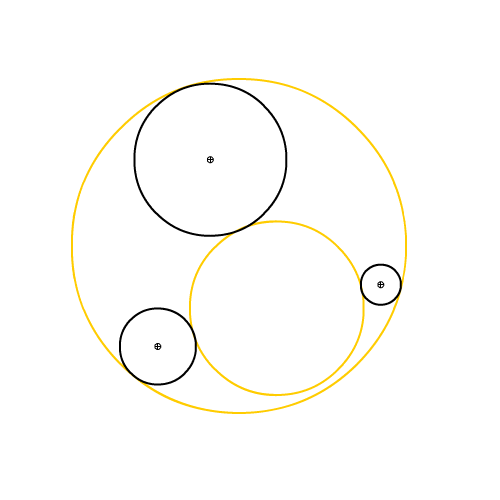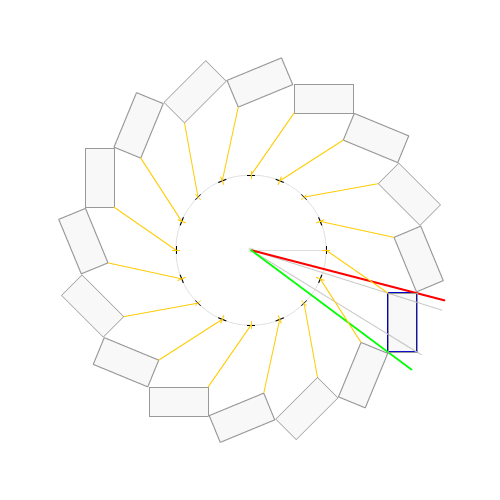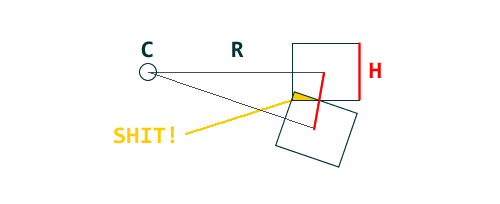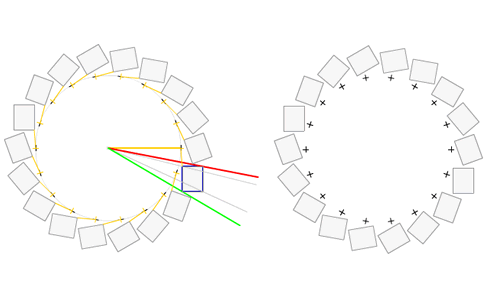# B.I.G.A. 8 : TOOLS FOR CIRCLEnow that we have a decent amount of tools to process lines, segments and triangles, it’s time to start using a paradoxically complex shape: Mister Circle.
Read the rest of this entry »

# distributing non overlapping objects on a circle

on May 21st, 2010
about: actionscript 3, geometry, graphics
feat. , , ,here’s a snippet to distribute shapes on a circle no matter how their regPoint is set.
not revolutionary but it can help :)
the tricky part is to determine how much space is taken by a given displayObject on the circle.
once you know that, you can create an ‘step angle’ to distribute your X shapes on a circle.
unfortunately, the step is not equal to the clip’s height:so you can’t just make it with a :

`clipCount = 2 * PI * r / clip.height`

instead you have to choose the lowest / highest angles to the center among the clip’s rect corners.
which gives us this little function:

```public function getCount( center:Point, clip:DisplayObject ):uint
{

var angle:Number = getAngle( center, new Point ( clip.x, clip.y ) );

var rect:Rectangle = clip.getBounds( clip );
rect.x += clip.x;
rect.y += clip.y;

var tl:Point = new Point( rect.x, rect.y );
var tr:Point = new Point( rect.x + rect.width, rect.y );
var br:Point = new Point( rect.x + rect.width, rect.y + rect.height );
var bl:Point = new Point( rect.x, rect.y + rect.height );

var corners:Array = [ tl, tr, br, bl ];

var min:Number = Number.POSITIVE_INFINITY;
var max:Number = Number.NEGATIVE_INFINITY;
for each( var p:Point in corners )
{
angle = getAngle( center, p );
if ( angle < = min ) min = angle;
if ( angle >= max ) max = angle;
}

return uint( Math.PI * 2 / ( max - min ) );

}
private function getAngle( p0:Point, p1:Point ):Number
{
return Math.atan2( p1.y - p0.y, p1.x - p0.x );
}```

to the left is the calculation, to the right the result.you see that even if the regpoint ( the cross) is not centered or left aligned, it still works.
to distribute the shapes on a circle, you’ll do something like:

```var count:int = getCount( center, clip );
var step:Number =  ( Math.PI * 2 / count );
var angle:Number;
var i:int;
for ( i = 0; i < count; i++ )
{
var c:Clip =  new Clip();
addChild( c );

angle = step * i;
c.x = center.x + Math.cos( angle ) * radius;
c.y = center.y + Math.sin( angle ) * radius;
c.rotation =  angle * 180 / Math.PI;
}```

where clip is the clip you want to distribute ( the distance between center and clip being taken as the radius ).
here’s a little demo to help understand better:

enjoy :)

© 2009 HIDIHO!. All Rights Reserved.

This blog is powered by Wordpress and Magatheme by Bryan Helmig.